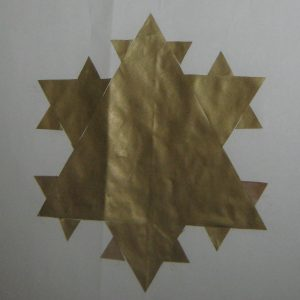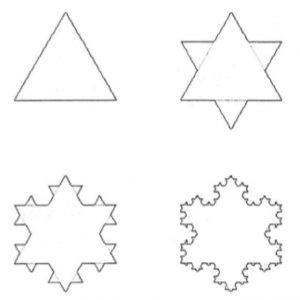The curve, also known as the ‘snowflake curve’, was invented in 1904 by the Swedish mathematician Helge von Koch.

Take a large piece of backing paper, and either draw an equilateral triangle with edges of length 27 cm or cut a triangle from coloured paper and stick it on a backing sheet.

Step 1       Divide the triangle edge length by 3.

Step 2       Make a triangle with this edge length for each edge of the previous shape. How many triangles do you need?   Record this number each time you go through these instructions.

Step 3       Stick one new triangle to the middle of each of the edges of the previous shape.

Repeat Steps 1 to 3 until you have made triangles that are 1cm edge length, and stuck them on.Imagine these steps being repeated again and again many times even though the triangles would actually get too small to handle.

The diagram shows Stages 0, 1, 2 and 3 of the von Koch curve, not fractals but steps in the creation of the fractal that would result if the process were to be repeated infinitely often. The outer edge of the snowflake pattern is called the von Koch curve.

The poster above is at Stage 2.

Record the lengths of edges you have made.

Can you predict what the lengths of edges of the next few sets of triangles would be?  [It is better to use fractions rather than decimals].